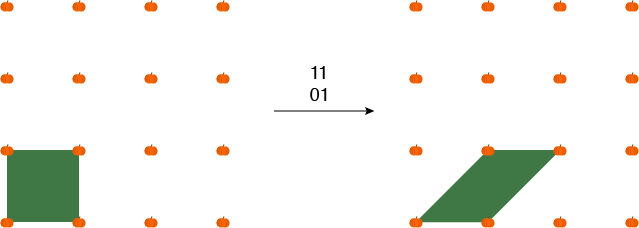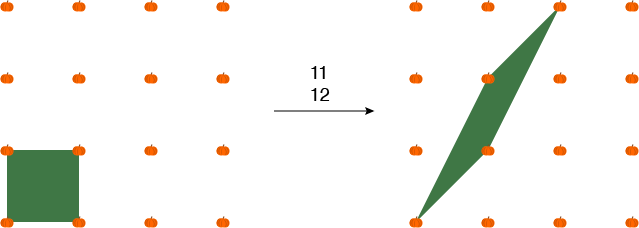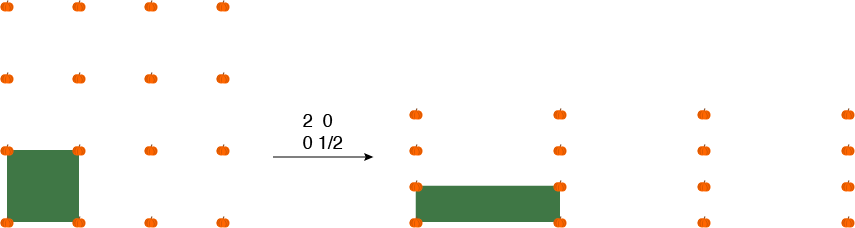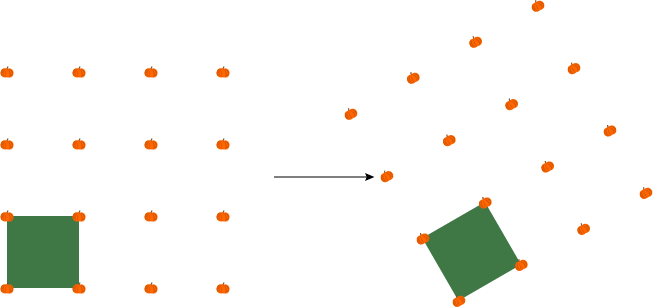I was a little confused a few years ago when I first saw social media posts and memes about PSL season. I don't drink coffee, so I was oblivious to the rise of the pumpkin spice latte. I’m in a bit of a math bubble sometimes, but even I didn’t think a large swath of the population was suddenly obsessed with projective special linear groups just because it was fall.

Did I write this post entirely because of coincidental initials? Yes. But these mathematical PSLs are close to my heart, so now that the aspens are turning gold and there’s a chill in the air, I’m going to take full advantage of this punny excuse for writing about projective special linear groups.

In mathematics, a group is a collection of mathematical objects along with a way to combine them to get other objects still in the same group. An easy example of a group is the set of integers with the operation of addition. When you add two integers, you get another integer. (There are a couple other technical requirements, but that’s the main idea.)

The L in the mathematical PSL means linear, which is a special type of transformation. These transformations can be represented by matrices, which are arrays of numbers that describe how the transformation acts.

A mathematician, like an Instagrammer clad in yoga pants, would not just order a PSL; she would specify what kind of PSL she wants. At the coffee shop, you might say you’d like it with almond milk, an extra shot of espresso, and a small amount of sweetener. In math, you’ll specify the dimension of your matrices and what kind of numbers they contain.

In my research area in graduate school, two PSLs were the most popular: PSL(2,Z) and PSL(2,R). The 2 means the matrices have two rows and two columns. The Z represents integers, so a matrix in PSL(2,Z) must have integer entries. The R represents real numbers, so a matrix in PSL(2,R) can have any real numbers, including fractions and irrational numbers like π, as entries.

We’ve gotten through the L of PSL. The S stands for special, which means the transformation does not distort areas. At this point, it might be good to look at an example. Below is an illustration of what happens to a square that is transformed by the matrix

 1 1 0 1

Because it is PSL season, I am of course illustrating with pumpkins. There is a pumpkin at each point with integer coordinates, making a nice little pumpkin (spice) lattice. The square we’re looking at is a little green patch between four pumpkins. You can think of it as having the point (0,0) for its bottom left corner. After the transformation, the resulting figure still has straight sides and the same area, but it’s not a square anymore.An illustration of the way a matrix acts on a square. Credit: Evelyn Lamb

A slightly more exciting matrix, also in PSL(2,Z), is

 1 1 1 2This matrix stretches the square further up than the previous matrix. Credit: Evelyn Lamb

The grassy patch between the pumpkins is distorted even more under this transformation, but it still has the same area as the original square. Luckily,you don’t have to measure the areas of strange regions to figure out whether your 2x2 matrix is special. If the matrix

 a b c d

is special, the quantity ad-bc, known as the determinant, is equal to 1.

When we leave PSL(2,Z) and move to PSL(2,R), we can have matrices like

 2 0 0 1/2This matrix makes long, skinny pumpkin patches. Credit: Evelyn Lamb

which stretches and (big wink) squashes not only the square but also the pumpkin lattice as well, or

 √3/2 -1/2 1/2 √3/2

which rotates the square and the lattice.Credit: Evelyn Lamb

Now a confession: what I’ve written so far is true for the groups SL(2,R) and SL(2,Z), but we haven’t talked about the P yet. P stands for projective, which means we identify opposites with each other. So the matrix

 1 1 0 1

is considered to be the same as the matrix

 -1 -1 0 -1

This identification makes it a little bit of a fudge to talk about a matrix in PSL(2,R). If you try to imagine PSL(2,R) as a space, then each “point” of the space is a pair of matrices, not a single matrix. So I’ve been implicitly choosing to think about one of the two matrices rather than both of them.

That brings us to the real utility of PSL(2,R) and PSL(2,Z) in my grad school research area. When I worked with these groups, it wasn’t because I was thinking about particular matrices acting on the plane. Instead, I cared about PSL(2,R) because of its relationship with the hyperbolic plane, one of the central objects in my research. One important tool for studying a space is its isometry group, the set of transformations that preserve distances in the space. In the regular Euclidean plane, translations and rotations are examples of isometries. There is a correspondence between the isometries of the hyperbolic plane and elements of PSL(2,R). For PSL(2,Z), there is a correspondence between elements of that group and different shapes of tori, surfaces with one hole.

One of my favorite quotes about mathematics is by Henri Poincaré, who said “mathematics is the art of giving the same name to different things.” Often the real work of mathematics is in finding the the right way to give the same name to different things. In the case of these two PSLs, that correspondence gives us a solid foundation to build on in our understanding of surfaces. As for the other PSLs, I’m afraid Poincaré didn’t say much about coffee, but another mathematician, Alfred Renyi did. He said (though this quote is often attributed to Paul Erdős) that “a mathematician is a device for turning coffee into theorems.” I think a reasonable corollary for fall is that a mathematician who drinks PSL must be turning it into theorems about matrices and surfaces.